# Images UPSC Questions

## Images MCQ Questions

1.

Consider the following matrix with one empty block in the lower extreme corner: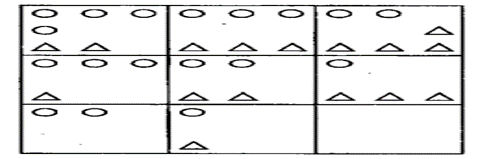Which of the following figures could fit in the empty block and thus complete the matrix?

A.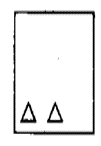B.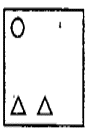C.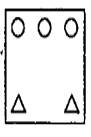D.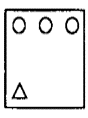A.2.

Consider the following figures: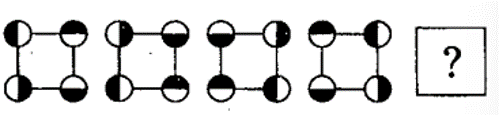Change in positions of beads in the four figures above follows a sequence. Following the same sequence, which of the figures below should appear as the fifth figure above?

A.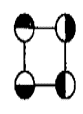B.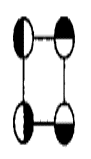C.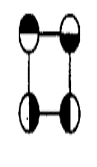D.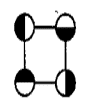B.3.

Consider the figures given below: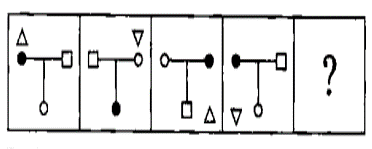To fit the question mark, the correct answer is

A.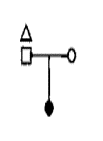B.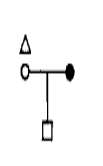C.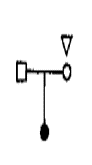D.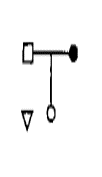A.4.

Examine the following figure: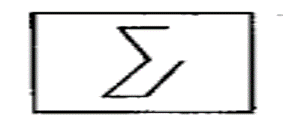Which one of the following figures has the above figure embedded in it?

A.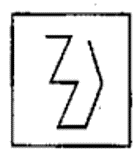B.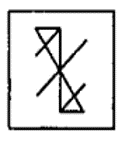C.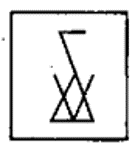D.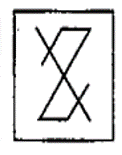C.5.

Consider the following matrix: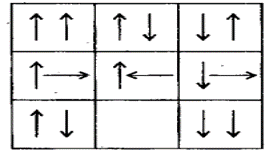Which one of the following figures fits into the blank part of the above matrix?

A.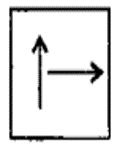B.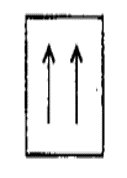C.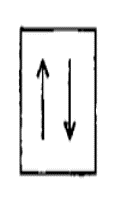D.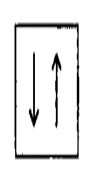C.6.

Consider the following figures 1, 2, 3 and 4 :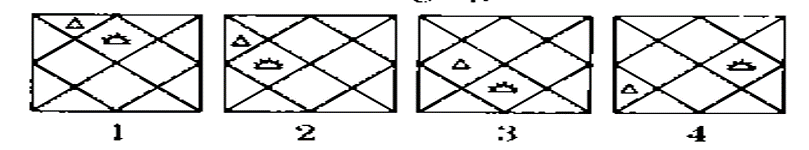In the figures from 1 to 4 above, two symbols are shown to change their position in a regular direction. Following the same sequence, which one of the following will appear at the fifth stage?

A.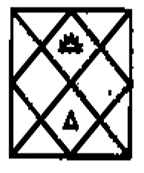B.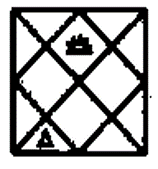C.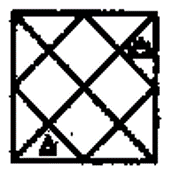D.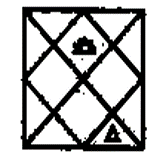B.